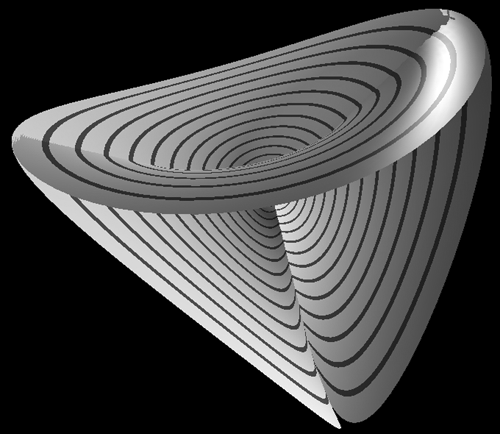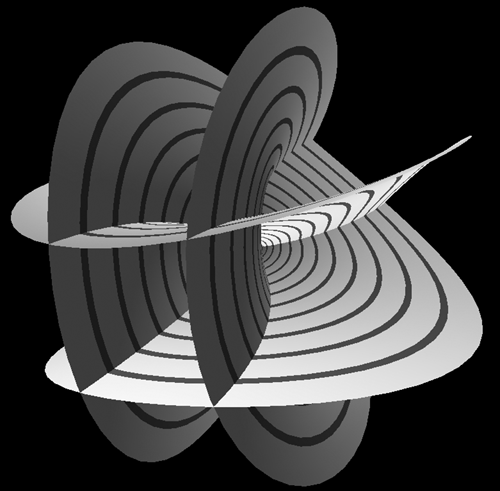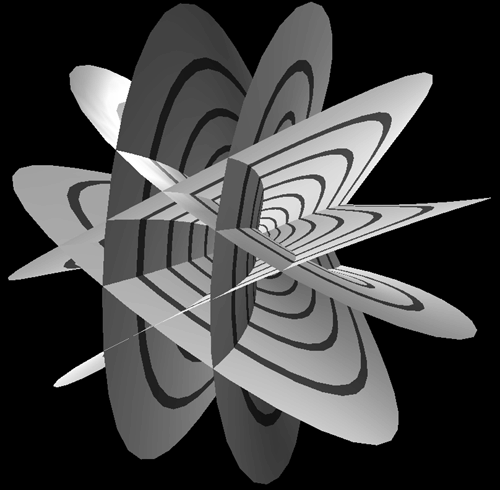# Bour Minimal Surface

Attributed to Edmond Bour
Equations by Roger Bagula

Graphics by Paul Bourke

February 2003

x = rn-1 cos((n-1) t)/(2 (n-1)) - rn+1 cos((n+1) t)/(2 (n+1))

y = rn-1 sin((n-1) t)/(2 (n-1)) + rn+1 sin((n+1) t)/(2 (n+1))

z = rn cos(n t) / n

0 <= r, 0 <= v <= 2 pi

n = 3n = 4n = 5n = 6